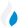•Trade Pi
•Trade
•Exchange
•Trade Pi
•Trade
•Exchange
\$1.09T
Total marketcap
\$79.33B
Total volume
41.05%
BTC dominance
•Trade Pi
•Trade
•Exchange

# kunci to IDR Exchange Rate - 1 Kunci Coin in IDR

270
Vol [24h]
\$25,385.64

## kunci to IDR converter

Exchange Pair Price 24h volume
Indodax KUNCI/IDR \$0.01803 \$25.65K

## KUNCI/IDR Exchange Rate Overview

Name Ticker Price % 24h 24h high 24h low 24h volume
Kunci Coin kunci \$0.01791 -1.704% \$0.01824 \$0.0178 \$980.44K

Selling 1 Kunci Coin kunci you get 270 IDR.

Kunci Coin Mar 4, 2022 had the highest price, at that time trading at its all-time high of \$0.9321.

332 days have passed since then, and now the price is 1.93% of the maximum.

Based on the table data, the KUNCI vs IDR exchange volume is \$25,385.64.

Using the calculator/converter on this page, you can make the necessary calculations with a pair of Kunci Coin to IDR.

## Q&A

### What is the current KUNCI to IDR exchange rate?

Right now, the KUNCI/IDR exchange rate is 270.

### What has been the Kunci Coin to IDR trading volume in the last 24 hours?

Relying on the table data, the Kunci Coin to IDR exchange volume is \$25,385.64.

### How can I calculate the amount of IDR? / How do I convert my Kunci Coin to IDR?

You can calculate/convert IDR from KUNCI to IDR converter. Also, you can select other currencies from the drop-down list.

## KUNCI to IDR Сonversion Table

KUNCI IDR
30 KUNCI = 8,100 IDR
60 KUNCI = 16,200 IDR
200 KUNCI = 54,000 IDR
200 KUNCI = 54,000 IDR
300 KUNCI = 81,000 IDR
600 KUNCI = 162,000 IDR
900 KUNCI = 243,000 IDR
3000 KUNCI = 810,000 IDR
6000 KUNCI = 1,620,000 IDR
60000 KUNCI = 16,200,000 IDR
600000 KUNCI = 162,000,000 IDR
6000000 KUNCI = 1,620,000,000 IDR
60000000 KUNCI = 16,200,000,000 IDR